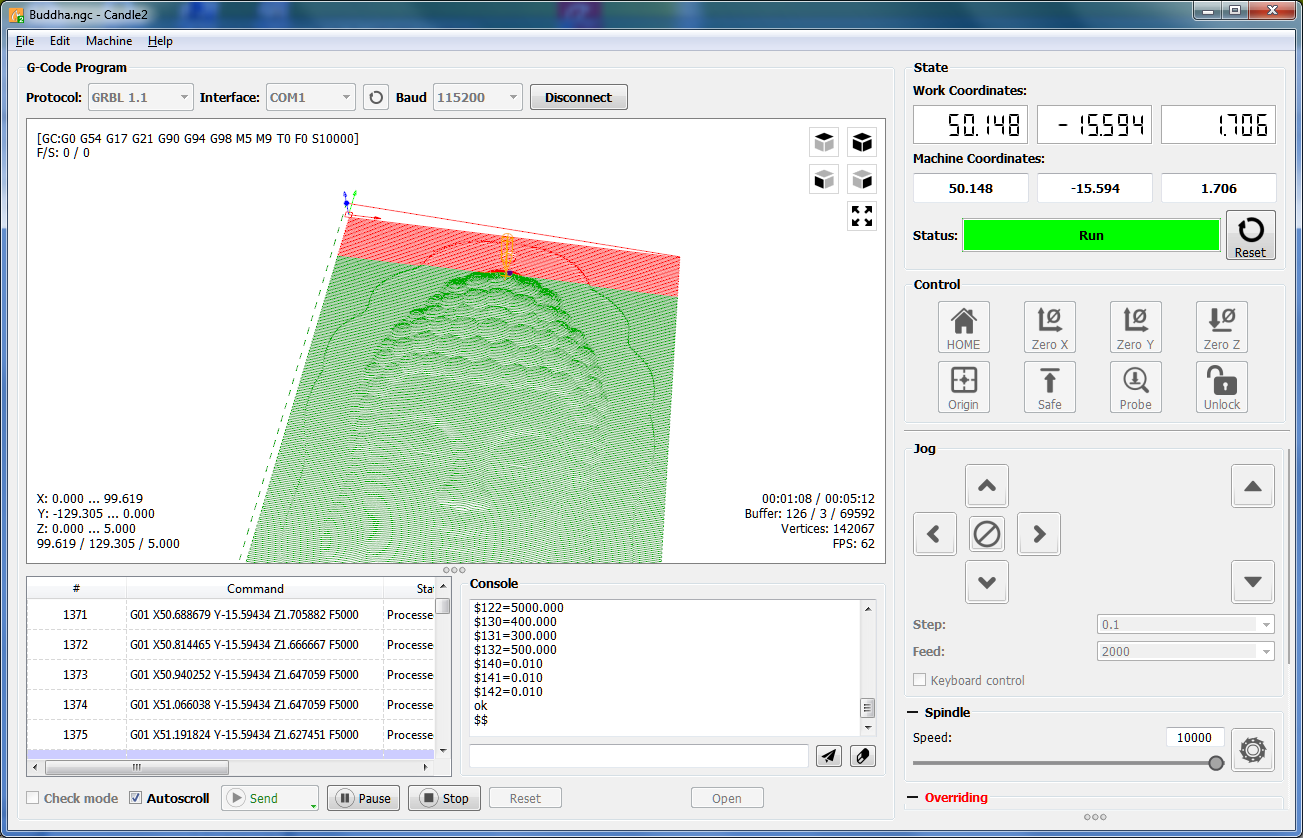开源改变世界!!

打开了这个问题 on 15 Oct 2019 · 9 条评论

# Candle 2 或 Grbl 板无响应或不稳定进给率高表示 > 2500#2

打开了这个问题 on 15 Oct 2019 · 9 条评论

## 注释

### klchin66 评论 on 15 Oct 2019

 你好， 您是否遇到过 Candle 无法与 Grbl 板同步， 在 Windows 操作系统上进给率很高时说 2500 或大约？Candle 可以响应 GUI 按钮，如复位按钮，但没有来自 GRBL 板的反馈。 如果使用 Override Feed rate，问题会发生得更快。说GCode Feedrate是 1000，override rate是1500左右的150%。在发送GCode期间的这种情况下， 如果尝试调整Candle的窗口大小问题会更快发生。 “缓冲区：”总是在 0/0/0，就像无法及时将 GCode 行推送到 GRBL 板上一样。 在进给率 5000 下使用 UGS 平台 V 2.0 夜间构建测试相同的设置也没有问题。 问候， 吉隆坡

### 席德克鲁特 评论 on 16 Oct 2019

 您好， 您使用的是哪个版本的 grbl？ 您可以发布您的 \$\$ 设置吗？ 你能在发生这种行为的地方附加一个 gcode 文件吗？

### klchin66 评论 on 16 Oct 2019

 你好， 我已经用https://github.com/thomast777 – F46 版本进行了测试， 如果没记错的话，你的 Grbl-A​​dvanced 版本也 与 stm32F407ZGt 有同样的问题。 我已将附件文件的进给率修改为 F5000 进行测试。 Buddha.zip 这是其中一个示例文件。 这是我昨天测试的设置。 \$1=1 \$2=0 \$3=1 \$4=0 \$5=0 \$6=1 \$10=1 \$11=0.010 \$12=0.002 \$13=0 \$20=0 \$21=1 \$22=1 \$23=3 \$24=100.000 \$25=1000.000 \$26= 250 \$27=5.000 \$30=5000 \$31=0 \$32=0 \$40=10000.000 \$100=8000.000 \$101=8000.000 \$102=8000.000 \$103=8000.000 \$104=8000.000 \$105=8000.000 \$110=8000.000 \$111=8000.000 \$112=8000.000 \$113=8000.000 \$114=8000.000 \$115 =8000.000 \$120=400.000 \$121=400.000 \$122=400.000 \$123=400.000 \$124=400.000 \$125=400.000 \$130=150.000 \$131=150.000 \$132=150.000 \$133=150.000 \$134=150.000 \$135=0.051 问候， 吉隆坡

### klchin66 评论 on 16 Oct 2019

 额外信息…它发生在 115200 或 256000。

### klchin66 评论 on 16 Oct 2019

 确认 Grbl-A​​dvanced 上的设置也有同样的问题。 这里的屏幕截图，它几乎发生在同一 GCode 行上（测试了 3 次）。这里是我测试的设置。 \$1=50 \$2=0 \$3=0 \$4=0 \$5=0 \$6=0 \$10=1 \$11=0.010 \$12=0.001 \$13=0 \$14=0 \$20=0 \$21=1 \$22=1 \$23=0 \$24=50.000 \$25= 500.000 \$26=250 \$27=1.000 \$30=3000 \$31=0 \$32=0 \$100=890.000 \$101=890.000 \$102=890.000 \$110=5000.000 \$111=5000.000 \$112=5000.000 \$120=5000.000 \$121=5000.000 \$122=5000.000 \$130=400.000 \$131=300.000 \$132 =500.000 \$140=0.010 \$141=0.010 \$142=0.010 还行

### klchin66 评论 on 16 Oct 2019

 刚刚注意到总是停在第 1375 行和错误：轴命令冲突。

 我会调查这个

 任何进展 ？

### 席德克鲁特 评论 on 6 Nov 2019

 有点…我在我的 ubuntu 机器上做了几次测试。完全没有问题，一切都运行得很完美。 但是你应该降低你的小数精度。点后 3 位数就足够了。它将提高性能。到现在为止 grbl 几乎所有的时间都花在解析 gcode 上，因为它包含了太多的数字。

### klchin66 评论 on 6 Nov 2019

 了解，也可能是操作系统问题。 顺便说一句，我使用 I7 HQ 4700 2.4Ghz 8G、NVidia 750M、Win7 Ultimate，它应该足以 处理 Gcode 解析器。 我注意到该应用程序花费大量时间处理 GUI 更新，例如更新状态和控制按钮。不知道 Qt 如何处理 GUI，我确实对 C# 处理多线程 GUI 有不好的体验。